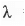# Points to be Remembered: Atomic Structure Notes - Chemistry

## Chemistry: Points to be Remembered: Atomic Structure Notes - Chemistry

The document Points to be Remembered: Atomic Structure Notes - Chemistry is a part of Chemistry category.
All you need of Chemistry at this link: Chemistry

1. The mathematical expression of radius of H-like atom is: r„ = n2h2/47π2mkZe2 = 0.529 A0, where n = no. of Bohr orbit, h = Planck's constant, m = mass of electron, Z = nuclear charge and e = charge of electron.
2. The mathematical expression of total energy of H- like atom is: En = -[2π2mk2Z 2e4]/[n2h2] = -13.598 eV Total energy is composed of kinetic energy (T) and potential energy (V).T he expression of kinetic energy and potential energy are: T = [2π2mk2z2e4 ] / [ n2h2] = E„ and V = - [4π2mk2Z2e4]/[n2h2] = -2En where n = no. of Bohr orbit, h = Planck's constant, m = m ass o f electron, Z = nuclear charge and e = charge o f electron.
3. When an electron is at infinite distance from the nucleus , there will be no interaction between the nucleus and the electron. Hence, the energy of the electron is assumed to be zero. But, when the electron is approaches towards the nucleus, there will be an attractive interaction between the positively charged nucleus and the negatively charged electron. Hence, the electron will get some stability. Hence, it will release some energy. Hence, its energy will become negative.
4. According to Bohr electrons can move only those orbit in which the angular momentum (mvr) is an integral multiple of h/2π i.e. mvr = nh/2π, where m = mass of electron, v = velocity of electron, r = radius of orbit, n = no. of orbit and h = Planck's constant.
5. According to de Broglie: X = h/mv, where X = wavelength, h = Planck's constant, m = mass of electron and v = velocity o f particle.
6. If V is the potential difference, the relation between the wavelength and potential difference is:= h/√[2eVm], where X = wavelength, e = charge of electron, V = potential difference, m = mass of electron.
7. According to Heisenberg : Δx.Δpx≧ h/4π, where Δx = uncertainty in position, Δpx = uncertainty in momentum and h = Planck's constant.
8. Quantum nos. can be classified into four types - principal quantum no. (n), azimuthal or, orbital quantum no. (1), magnetic quantum no. (m) and spin quantum no. (s). Principal quantum no. determine the energy of an electron. Azimuthal quantum no. determine the shape of an orbital. Magnetic quantum no. determine the magnetic orientation of orbital. Spin quantum no. determine the spin of electrons.
9. The no. of radial nodes, angular nodes and maxima are : (n-1-1), 1 and (n-1) where n and 1 are principal and azimuthal quantum nos.
10. Rules for finding out the ground state term symbol:

➤ Find out the spin multiplicity (2S+1) first.

➤ Then find out the orbital multiplicity (L).

➤ Next determine the J value. J = |L + S | to | L - S j. Highest and lowest J values are taken for more than half-filled and less than half-filled electronic configurations.

➤ Finally, the GT symbol is : (2S+1)Lj
11. Rules for symmetry allowed atomic transitions:

➤ The change in the azimuthal quantum nos. will be ±1 i.e. A1 = +1.

➤ The change in the spin will be 0 i.e. As = 0.

The document Points to be Remembered: Atomic Structure Notes - Chemistry is a part of Chemistry category.
All you need of Chemistry at this link: ChemistryUse Code STAYHOME200 and get INR 200 additional OFF

Track your progress, build streaks, highlight & save important lessons and more!

,

,

,

,

,

,

,

,

,

,

,

,

,

,

,

,

,

,

,

,

,

;